# Thermal-Electrical Analogy for Dynamic Urban-Scale Energy Modeling

Thermal-Electrical Analogy for Dynamic Urban-Scale Energy Modeling
Department of Energy, R3C, corso Duca degli Abruzzi 24, Torino10129, Italy

Department of Energy, FULL, corso Duca degli Abruzzi 24, Torino 10129, Italy

Department of Energy, corso Duca degli Abruzzi 24, Torino 10129, Italy

Corresponding Author Email:
guglielmina.mutani@polito.it
Page:
571-582
|
DOI:
https://doi.org/10.18280/ijht.380301
20 June 2020
|
Accepted:
30 July 2020
|
Published:
15 October 2020
| Citation

OPEN ACCESS

Abstract:

The development of urban-scale energy modeling is currently the goal of many research groups as a result of to the increased interest in evaluating the impacts of energy efficiency measures in cities. These energy models are useful to explore consumption and emission distribution at district scale and to quantitatively assess renovation strategies and energy supply options.

This work presents a dynamic energy modeling based on an energy-balance of residential buildings which uses urban-scale data. The model was designed to take into account the microclimate conditions and morphological urban-scale parameters. This methodology was calibrated, optimized and validated by applying it to a district in the city of Turin.

In addition, a thermal-electricity analogy has been implemented in a software for scientific computation and simulation to manage big database. Therefore, a new model based on thermal-electrical analogies, which can be applied to a larger building stock, is presented. A sensitivity analysis has highlighted the different types of behavior of building considering their periods of constructions. The results of this work show that this model can also be applied at a district scale with good accuracy, especially for old buildings, to manage the existing large databases for analyses at an urban or territorial scale; for newer buildings needs to be improved.

Keywords:

building energy balance, hourly model, residential buildings, urban scale, urban variables, thermal-electrical analogy, place-based analysis

1. Introduction

A reduction in energy consumption in buildings could be one of the main drivers considered to improve the energy sustainability and quality of urban environments, in order to exploit the production of energy from the available renewable sources .

In this context, the urban-scale energy models (USEM) are useful to explore the consumption, production and productivity of buildings at a territorial scale in order to achieve the energy security [2, 3]. Since the relationship between urban form and buildings affects the energy performances of buildings, these models can be used to obtain a lower energy demand by improving the morphology of the built environment . So far, USEMs have only considered few variables that are known to influence consumption, especially as regards the urban context.

The novelty of the energy model presented in this work is that a number of urban variables have been added to the energy balance to take into account the surrounding context: solar exposition, urban canyon height-to-distance ratio, characteristics of outdoor spaces, and the presence of vegetation .

Recently an ‘engineering’ model was developed for the city of Turin (IT), with the support of Geographical Information System and Google Earth tools, in order to create an urban energy atlas for the building stock . In this work, that model has been improved by moving from monthly to hourly consumption data and introducing a thermo-electrical analogy.

The aim of this work has been to create a platform that may be used by stakeholders, energy producers and distributors, urban planners and policy makers to plan smart energy systems and sustainable cities, and to obtain information, at different scales, about energy consumption, production and emissions. With this engineering model, it will also be possible to evaluate how energy consumption changes according to the energy efficiency level and system efficiency of a building, but also according to urban morphology . The goal of this platform is to guarantee energy security, affordability and environmental sustainability for more resilient cities [7, 8].

2. Material and Methods

This work introduces an hourly urban-scale energy model of residential buildings together with a thermal-electricity analogy.

The used methodology is based on the existing ISO 52016-1:2017 and ISO 52017:2017 standards which describe the energy balance equations that can be applied to a single building. In this work, these equations have been simplified considering the available buildings data for the city of Turin, using existing georeferenced databases such as technical maps, the reference territorial database, a digital territorial model, a digital surface model and data from the population and housing census for the year 2011. All cities have this data and therefore this analysis can be easily replicable. A GIS tool has been used to identify all the characteristics of the buildings that influence their consumption for space heating.

## 1.png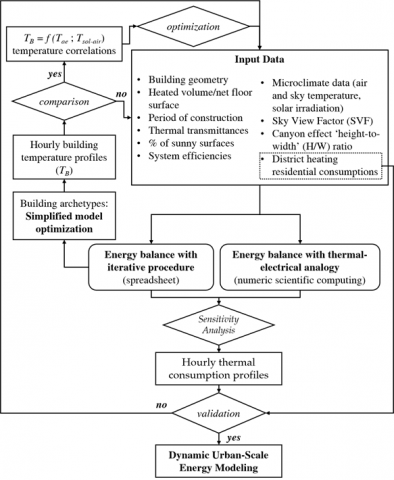Figure 1. Flowchart of methodology

The thermal-electrical model has been implemented with the support of Matlab considering the input data previously identified for the different building typologies and local climate conditions .

The accuracy of the energy balance model has been evaluated by comparing the calculated and measured energy consumptions for space heating on about 100 residential buildings in Turin for the 2013-14 and 2014-15 heating seasons. In the first part of the work, a daytime temperature of the buildings was set equal to the winter comfort conditions (i.e. 20 ± 2°C).

The building temperature was then corrected to reduce the model errors, considering the main local climatic variables that influence energy consumption. The accuracy of the model was improved with this optimization.

Figure 1 describes the methodology used to design the dynamic urban-scale energy model: the main input data; the iterative procedure; the identification of building archetypes by construction periods; the analysis of hourly temperatures profiles; the model optimization with the identification of correlations between the buildings temperature and the local climate conditions; the thermal-electrical analogy process; and the model validation.

2.1 Energy balance model

In the first part of the analysis, input data were selected considering the data available for residential buildings at an urban scale. Some missing variables, such as the solar shadings, the view factors and building orientations were calculated at a block of building scale and were then associated with each building.

Subsequently, each building was subdivided into three thermodynamic systems:

- the inside part of the building with air, internal partitions, furniture, people and objects: called B – building;

- the opaque envelope that separates the inside part of the building from the outside, unheated and other heated environment: called E – envelope;

- the transparent elements that separates the inside part of the building from the outside environment: called G – glazing.

This model has concentrated parameters and the three thermodynamic systems exchange heat with each other. Moreover, the heat supplied to the building is known and there are three equations for the three unknowns that are the temperatures of the three thermodynamic systems (TS).

The energy balance equation used for the three TSs is the following:

$C_{T S} \frac{d T_{T S}}{d t}=\emptyset_{\text {sol}}+\emptyset_{I}+\emptyset_{H}-\left(\emptyset_{T}+\emptyset_{V}\right)$     (1)

where: C is the heat capacity [JK-1], T is the temperature [K], t is the time [s], ϕsol is the heat flow rate from solar gains [W], ϕI is the heat flow rate from internal gains, ϕH is the heat flow rate from the heating system, ϕT is the heat flow rate dispersed by transmission, ϕV is the heat flow rate dispersed by ventilation.

The three energy balance equations were solved for each hour, through an iterative method, by setting a maximum number of 100 iterations and an acceptable error of 0.001.

The main assumptions on which this model is based are as follows:

1. the buildings are thermal zones with a uniform temperature,
2. the heat flow is unidirectional (and thermal bridges were neglected) and is calculated through a heat exchange coefficient,
3. the latent heat for humidification or dehumidification was neglected because residential buildings in Italy have water heating systems (the relative humidity of the air is not controlled)
4. at daytime in the buildings, the comfort thermal conditions are reached.

The input data and the variables used for the energy balances of the three thermodynamic systems described with Eq. (1) are introduced in the next subsections.

2.1.1 Heat flow rate from solar gains ϕsol

The solar heat flow depends on: the characteristics of solar absorbance, reflectance and transmittance characteristics of the opaque and transparent building elements, the sunny areas of the building envelope and the incident solar irradiance.

The solar heat flow can be divided into two components:

(1) the heat flow absorbed by the opaque and transparent envelope element k:

$\emptyset_{s o l, \alpha}=\sum \alpha_{k} \cdot I_{s o l} \cdot \xi \cdot F_{k} \cdot A_{k}$     (2)

(2) the heat flow that enters the building through the envelope element k:

$\emptyset_{\text {sol}, \tau}=\sum \tau_{G} \cdot I_{\text {sol}} \cdot \xi \cdot F_{k} \cdot A_{k}$     (3)

The solar absorbance α and total transmittance τ depend on the type of opaque and transparent envelope while the solar irradiance Isol depends on the climate conditions recorded at the weather stations. Area A of element k was calculated with a GIS tool, using the aforementioned technical map and the digital surface model. In order to evaluate the share of sunny envelope ξ, the solar height ψ was calculated using solar geometry:

$\psi=\sin ^{-1} \cdot(\sin \varphi \cdot \sin \beta+\cos \varphi \cdot \cos \beta \cdot \cos z)$     (4)

$a=\sin ^{-1} \cdot(\cos \beta \cdot \sin z / \cos h)$     (5)

$\beta=\sin ^{-1} \cdot\{0.398 \cdot \sin [0.9863 \cdot(d-82)]\}$     (6)

$\xi=\left\{\begin{array}{lll}\frac{\tan (\psi)}{H / W} & \text { if } & h<\arctan (H / W) \\ 1 & \text {if } & h \geq \arctan (H / W)\end{array}\right.$     (7)

The solar height ψ was evaluated for each hour using Eqns. (4), (5) and (6) and knowing: latitude $\varphi$, solar declination β, hour angle z = 15 (sh-12), solar hour sh and day number d. The share of the sunny part of the envelope ξ was then evaluated with Eq. (7) knowing the urban canyon geometry (height-to-width ratio H/W).

The effective share of the sunny part of the envelope takes into account the obstruction factor Fk which was evaluated with the sky view factor (SVF) utilizing the Relief Visualization Toolbox.

2.1.2 Heat flow rate from internal gains ϕI

The internal gains of residential buildings can be evaluated from the average floor area of dwellings Sf and the number of dwellings n:

$\emptyset_{I}=q_{i n t} \cdot S_{f} \cdot n$     (8)

while the hourly profiles of qint due to occupants and equipment for daytime and nighttime in residential buildings was derived from the UNI/TS 11300-1:2014 and ISO 13790:2008 standards.

2.1.3 Heat flow rate from the heating system ϕH

The heat flow rate from the heating system was calculated from the energy ϕS,H delivered by the district heating company to the buildings every hour and from the efficiency of the heating system ηH:

$\emptyset_{H}=\emptyset_{S, H} \cdot \mathrm{n}_{H}$     (9)

The hourly energy consumptions were calculated by linear interpolation from the cumulative consumptions in order to derive the exact hourly data. The heating in the analyzed buildings is shutdown at night from 10 pm to 5 am and it was assumed, in the thermal model, that once the comfort temperature has been reached, the system shuts down.

2.1.4 Heat flow rate by transmission ϕT

The heat flow dissipated by transmission, $\emptyset_{T}$, through the opaque and transparent envelope towards the outside environment (or other unheated environments) depends on area A, the thermal resistance R of the dispersing elements and the temperature gradients:

$\emptyset_{T, t}=\sum \frac{A_{k}}{\frac{1}{2} \cdot R_{k}+R_{s e}} \cdot b \cdot\left(T_{T S}-T_{a e}\right)-$$\sum \frac{A_{k}}{\frac{1}{2} \cdot R_{k}+R_{s i}} \cdot\left(T_{T S}-T_{B}\right) . \emptyset_{H}=\emptyset_{S, H} \cdot \eta_{H}$    (10)

The correction coefficient b is used if the heat flow is dissipated by transmission to an unheated environment (b=1 to the external environment).

Moreover, the extra heat flow, due to thermal radiation lost to the sky ϕT,r by the building envelope, was added:

$\emptyset_{T, r}=\sum F_{r} \cdot R_{s e} \cdot U_{k} \cdot A_{k} \cdot h_{r, k} \cdot\left(T_{a e}-T_{s k y}\right)$     (11)

where: the form factor building-sky Fr was evaluated with the sky view factor (SVF) and the view factor (that depends on the control surface inclination); R and U are the thermal resistances and transmittances; hr is the radiative heat transfer coefficient between the envelope area A of element k and the sky.

2.1.5 Heat flow rate by ventilation ϕV

The heat flow dissipated by ventilation $\emptyset_{V}$ was estimated from the specific heat of the air $c_{a},$ the temperature gradient between the inside and outside air and the air mass flow rate $\dot{m}_{a}$ (knowing the air changes per hour ach , the air density and the air volume ):

$\emptyset_{V}=c_{a} \cdot \dot{m}_{a} \cdot\left(T_{a i}-T_{a e}\right)$     (12)

The ach, for residential buildings, can be estimated as 0.3-0.5 h-1 depending on the type of windows and on the maintenance status of the building .

2.2 Thermal-electrical analogy

The energy balance of the buildings was described by means of thermal-electrical analogy, using a scientific numerical computational program. This process is fundamental when the model is applied at an urban scale because it allows the consumption of a large number of buildings to be managed and calculated .

The thermal-electrical analogy process is based on the similarity shown by the equations that model the heat transfer among systems and the equations that model the electric current flow in a circuit [12, 13]. Like other analogies adopted in the field of engineering (i.e. hydraulic-electrical and electrical-mechanical-acoustical analogies), it allows the schematics of system to be drawn using well known international electric symbols Simulation programs, specifically developed for electric networks to be used to solve thermal system. Some tools already consider the thermal-electrical analogy, but at block of building scale, not at an urban scale. For example, CitySim is based on thermal-electrical analogy, where the heat flow between walls and the external air temperature can be represented by an electrical current through a resistor, and the inertia of wall is the capacitance .

If a mono-dimensional heat transfer is considered, the heat transferred to a thermal conductor is proportional to the difference in temperature at the end of the conductor by a proportionality constant that is equal to the thermal conductance (Y). Analogously, the electric current in an electric conductor is proportional to the voltage drop across its terminals, and the proportionality constant is equal to the electric conductance.

The heat flow in a body that is able to store energy is proportional to its temperature derivative by a constant, that is, the thermal capacity (C), while the electric current in a capacitor is proportional to the voltage derivative across its terminal, and the proportionality constant is equal to the electric capacitance.

Table 1. Electrical - thermal analogy: Components

Symbols

Voltage

Temperature

Current

Heat Flow

## t1.pngElectrical resistance; Electrical conductance time invariant

Thermal resistance; Thermal transmittance, time invariant

## t2.pngElectrical resistance; Electrical conductance; time dependent

Thermal resistance; Thermal transmittance, time dependent

## t3.png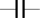Electrical capacity

Thermal capacity

## t4.pngIndependent voltage source

Independent temperature

## t5.pngIndependent current source

Assigned heat flow

A body with a fixed or assigned temperature can be assumed as an independent electric voltage source (T), while a heat flow can be modelized as an independent current source (I).

Starting from the equations that rule the thermal system, an electric network can be defined using the internationally defined electrical symbology and a thermal environment problem can be solved by solving an electric circuit, if the input parameters of the electric circuit have been set properly (Table 1).

The three thermal systems (G, E and B), with reference to Eq. (1), can be represented by an electric circuit with three parallel capacitors connected to three independent current sources, which represent the addition of heat to the system, and are interconnected by resistors. The energy balances used for the three thermodynamic systems are:

$C_{G} \frac{d T_{G}}{d t}=\sum \alpha_{G, k} \cdot I_{s o l, k} \cdot \xi_{k} \cdot F_{k} \cdot A_{G, k}-\sum \frac{A_{G, k}}{\frac{1}{2} \cdot R_{G, k}+R_{s e}} \cdot\left(T_{G}-T_{a e}\right)+$

$-\sum \frac{A_{G, k}}{\frac{1}{2} \cdot R_{G, k}+R_{s i}} \cdot\left(T_{G}-T_{B}\right)-\sum F_{r} \cdot R_{s e} \cdot U_{G, k} \cdot A_{G, k} \cdot h_{r, G, k} \cdot\left(T_{a e}-T_{s k y}\right)$     (13)

$C_{E} \frac{d T_{E}}{d t}=\sum \alpha_{W, k} \cdot I_{s o l, k} \cdot \xi_{k} \cdot F_{k} \cdot A_{W, k}-\sum \frac{A_{E, k}}{\frac{1}{2} \cdot R_{E, k}+R_{s e}} \cdot b \cdot\left(T_{E}-T_{a e}\right)+$

$-\sum \frac{A_{E, k}}{\frac{1}{2} \cdot R_{E, k}+R_{s i}} \cdot\left(T_{E}-T_{B}\right)-\sum F_{r} \cdot R_{s e} \cdot U_{W, k} \cdot A_{W, k} \cdot h_{r, W, k} \cdot\left(T_{a e}-T_{s k y}\right)$     (14)

$C_{B} \frac{d T_{B}}{d t}=\sum \tau_{G, k} \cdot I_{s o l, k} \cdot \xi_{k} \cdot F_{k} \cdot A_{G, k}+\emptyset_{\mathrm{I}}+\emptyset_{H}-\sum \frac{A_{E, k}}{\frac{1}{2} \cdot R_{E, k}+R_{s i}} \cdot\left(T_{B}-T_{E}\right)+$

$-\sum \frac{A_{G, k}}{\frac{1}{2} \cdot R_{G, k}+R_{s i}} \cdot\left(T_{B}-T_{G}\right)-c_{a} \cdot \dot{m}_{a} \cdot\left(T_{a i}-T_{a e}\right)$     (15)

An independent voltage source, which represents the external ambient temperature value, is also present in the circuit (Figure 2).

If the heat flow through ventilation, with variable infiltrations and window openings, is neglected, the electric circuit is a circuit with constant impedances (the resistor and capacitor values are not affected by the time) and with independent current and voltage sources that are time dependent. This kind of network is usual in electrical engineering and can be analyzed by considering the superposition effect: each generator in the circuit is considered individually and the currents/voltages produced by each generator are then summed.

If the heat flow through ventilation, with variable infiltrations and window opening, is considered, a time dependent resistance (or conductance) is introduced into the circuit. According to this assumption, the symbolic representation cannot be used, and the system requires the system of differential equations to be numerically solved.

The specificity of the electric circuit that is equivalent to the adopted thermal model (described with Eqns. (13), (14), (15) for the three thermodynamic systems G, E and B) is that the time variant parameter is the value of a conductance in a closed network, where the conductance itself is in series with an independent current source and a voltage source (Figure 2 and Table 2). This allows a simplified method to be adopted in the solution of the circuit.

## 2.pngFigure 2. The thermal-electrical analogy process

Table 2. Electrical model parameters and reference to Eqns. (13), (14), (15)

 Var. Thermal balance components $I_{G}$ $\sum \alpha_{G} \cdot I_{\text {sol}} \cdot \xi \cdot F \cdot A_{G}-\sum F_{r} \cdot R_{\text {se}} \cdot U_{G} \cdot A_{G} \cdot h_{r, G} \cdot\left(T_{\text {ae}}-T_{s k y}\right)$ $Y_{G, a e}$ $\sum \frac{A_{G}}{\frac{1}{2} R_{G}+R_{s e}}$ $Y_{G, B}$ $\sum \frac{A_{G}}{\frac{1}{2} R_{G}+R_{s i}}$ $I_{E}$ $\sum \alpha_{E} \cdot I_{s o l} \cdot \xi \cdot F \cdot A_{E}-\sum F_{r} \cdot R_{s e} \cdot U_{E} \cdot A_{E} \cdot h_{r, B} \cdot\left(T_{a e}-T_{s k y}\right)$ $Y_{E, a e}$ $\sum \frac{A_{E}}{\frac{1}{2} R_{E}+R_{s e}} \cdot b$ $Y_{E, B}$ $\sum \frac{A_{e}}{\frac{1}{2} R_{E}+R_{s i}}$ $I_{B}$ $\phi_{H}+\phi_{I}+\sum \tau_{G} \cdot I_{\text {sol}} \cdot \xi \cdot F \cdot A_{G}$ $Y_{B, ae }$ $c_{a} \cdot \dot{m}_{a}$

A common problem is the estimation of the energy consumption of a building, or a group of buildings, with assigned weather conditions, before it is (or they are) connected to the DH network. Weather conditions are generally non-period functions, but it is usual to predict the consumption by referring to standard days, which are defined considering the local climate conditions in the specific location. In this case, the consumptions were computed assuming that the local climate conditions were equal to a long series of reference days, thus the consumptions could be evaluated from the Fourier series and using the symbolic method.

Considering that there are thermal valves on each heat exchanger, which regulate the water flow in order to maintain the temperature in the living volume of the building at a constant value, as set by the user, the consumptions can be estimated in two steps.

The consumptions for an assigned indoor temperature can initially be evaluated without considering window ventilation. Then, considering that the time variant thermal conductivity is in a closed network series connected to an independent current source and a voltage source, the heat flow due to ventilation can easily be computed (the indoor temperature is an input value and the external temperature is a constraint). The additional consumptions following ventilation are then added to the previous value computed without considering ventilation.

In this way, a simple and quick algorithm that does not require numerical integration is used.

2.3 Optimization and validation

The model was validated by considering the different contributions to the energy balance equations and then comparing the consumptions calculated by the model with the measured consumptions. A comfortable building temperature of 20 ± 2℃ was assumed during the day (from 8 a.m. to 9 p.m.) to calculate energy consumption.

In order to improve the accuracy of the model results, it was assumed that the building temperature could vary depending on outdoor local climatic conditions and in particular on the sun-air temperature, which takes into account the contribution of air temperature and solar radiation.

3. Case Study

Turin is located in the northwestern part of Italy and it has a continental temperate climate. In Turin, residential buildings are mainly large and compact condominiums, and 80% of them were built before 1970 . In order to evaluate the accuracy of the hourly model on residential buildings, the model was applied to a neighborhood with about 100 residential buildings. These buildings described the typical characteristic of the building heritage of the city.

3.1 Buildings characteristics

Buildings can be described with geometrical and typological characteristics for energy performance evaluations. Geometrical characteristics were elaborated with a GIS tool from maps and territorial information. The thermo-physical characteristics of a building can be identified according to its period of construction (UNI/TR 11552, ).

In this work, some of the characteristics of the buildings in Turin were elaborated from a survey on more than 125,000 energy performance certificates (EPC) released in the 2009-2015 period for the city of Turin . The median values of the energy-related variables are reported in Table 3, where they are subdivided according to the periods of construction of the buildings. It is possible to observe that the level of efficiency of the considered buildings (with thermal transmittances U and EP) and heating systems (with heating system efficiencies ηH and EP) begins to improve for the buildings in the 1971-80 period and in particular for the last three construction period classes.

The results of an EPC survey on buildings after energy retrofitting interventions are reported in Table 4. Lower energy performance indexes (EP) and lower thermal transmittances (U) and higher values of system efficiencies (ηH) can be observed.

Table 3. EPC for generic reasons (median values)

 Period of construction n.EPC - Sn m2 Vg m3 S/V m2/m3 Uop W/m2/K Uw W/m2/K Ug W/m2/K ηH - EPh kWh/m2 EPgl kWh/m2 < 1919 19184 53.4 220.0 0.41 1.22 3.53 3.91 0.67 176.3 214.6 1919-45 21038 52.5 213.4 0.42 1.25 3.96 4.44 0.66 187.0 226.3 1946-60 37875 57.0 219.8 0.38 1.15 4.23 4.79 0.66 173.0 215.5 1961-70 27544 65.8 249.5 0.37 1.15 4.45 5.06 0.67 167.1 209.8 1971-80 5267 76.0 289.0 0.36 1.15 4.32 4.90 0.69 159.6 200.4 1981-90 1635 75.0 267.4 0.45 1.07 3.00 3.25 0.68 149.2 180.8 1991-05 4891 71.6 255.1 0.43 0.64 2.79 2.99 0.70 104.0 131.8 2006-15 3662 62.8 239.5 0.50 0.39 1.86 1.83 0.83 59.9 82.9

Table 4. EPC after retrofitting interventions (median values)

 Period of construction n.EPC - Sn m2 Vg m3 S/V m2/m3 Uop W/m2/K Uw W/m2/K Ug W/m2/K ηH - EPh kWh/m2 EPgl kWh/m2 < 1919 907 79.3 342.0 0.47 1.15 2.40 2.50 0.80 126.7 157.0 1919-45 738 78.6 329.5 0.48 1.14 2.66 2.82 0.80 136.4 164.2 1946-60 1368 70.3 282.8 0.48 1.08 2.96 3.20 0.78 133.3 165.9 1961-70 803 86.0 324.0 0.44 1.15 3.25 3.56 0.79 132.5 167.1 1971-80 122 155.8 582.7 0.50 1.09 3.08 3.35 0.79 134.1 155.3 1981-90 59 133.5 454.0 0.61 0.64 2.40 2.50 0.77 126.2 147.8 1991-05 88 88.4 292.6 0.55 0.90 2.80 3.00 0.78 102.7 124.7 2006-15 54 68.1 248.9 0.74 0.32 1.77 1.71 0.87 63.5 83.0

Table 5. Thermal capacities in Jm-2K-1 (per m2 of envelope area) for different periods of construction

 Elements and TS < 1945 1946-60 1961-70 > 1971 Slab above cellar 317,867 Attic slab 434,400 Walls 574,560 231,000 191,520 E (Envelope) 495,001 510,786 285,154 235,736 G (Glazing) 7,314 (single glass 4 mm) – 2x (double glass) B (Building) 30,496*

* the reference area [m2] is the net heated surface, and not the envelope area.

Table 6. Characteristics of the building sample

 Data 1919-45 1946-60 1961-70 1971-80 S/V ratio 0.28 0.27 0.28 0.35 Gross volume [m3] 6,986 5,892 5,968 12,364 Net heated floor [m2] 1,559 1,284 1,322 2,965 DH consumption [kWh/m3/y] 30.74 28.84 26.15 22.44 CE [kJ/K] 779,146 659,224 380,845 839,254 CG [kJ/K] 1,731 1,327 1,367 3,118 CB [kJ/K] 47,539 39,158 40,325 90,406 No. of dwellings 20 10 14 33 Heated surface of dwellings Sn/dw [m2/dw] 77 118 97 97

The heat capacity (C) of the typical envelope elements and thermodynamic systems as defined in UNI/TR 11552:2014 are shown in Table 5. The last rows in the table indicate the thermal capacities that can be adopted for the three thermodynamic systems. Only the internal part of the building consisting of the internal air, furniture and partitions is considered for the thermodynamic system B. This value was determined considering that the internal part of the building with the first 10 cm of envelope has a thermal capacity of 165 kJ/m2/K (UNI/TS 11300-1:2014).

These thermal transmittances, heat capacities and system efficiencies values were used to characterize the type of envelope and heating system in the components of the thermal balances in Eqns. (13), (14) and (15).

The results of the application of the thermal-electrical analogy to four typical buildings of different construction periods called “archetypes” are reported in this work. These buildings were chosen from among 92 buildings in a central high-density area in Turin with buildings connected to the district heating network (with night shutdown of the heating system). It can be seen, in Table 6, that these residential condominiums are compact buildings with low form factors and a decreasing heating consumption for newer buildings. This is obviously just an average consumption, because the presented model is only based on the period of construction of the buildings and the information on retrofitting interventions was obtained from the EPC database, which only mentioned 3.4% of the buildings in Turin.

The first part of the analysis consisted of an analysis of the consumption profiles of the buildings for typical heating season days. The daily consumption profiles for the four sample buildings are shown in Figure 3. A typical behaviour, already observed in other studies , is the heat peak at 6 a.m. and an almost constant daily consumption; moreover, the peak decreases as a function of the daily outdoor temperature (i.e. Figures 6a, 6b, 6c and 6d) and of the building efficiency level, which in this case depends on the period of construction.

## 31.png(a) Tae = 1.7℃

## 32.png(b) Tae = 4.3℃

## 33.png(c) Tae = 8.7℃

## 34.png(d) Tae = 12.5℃

Figure 3. Space heating consumptions (Wh/m3) varying Tae

4. Results and Discussion

This section presents the main results obtained from the application of the dynamic urban-scale thermal balance to four typical residential buildings selected according to the period of construction. For comparable results, it was chosen to represent the results with constant infiltration ventilation (ach = 0.5 h-1).

4.1 Heat flux components

The heat flow components of the energy balance of residential buildings were investigated to assess the contribution of: the heat flow rate released from the heating system (ΦH), the heat flow rate resulting from the internal heat sources (ΦI), the heat flow rate obtained from solar radiation (Φsol), the heat flow rate lost by transmission (ΦT,Eand ΦT,G), and the heat flow rate lost by ventilation (ΦV).

## 51.png(a) 1919-45

## 52.png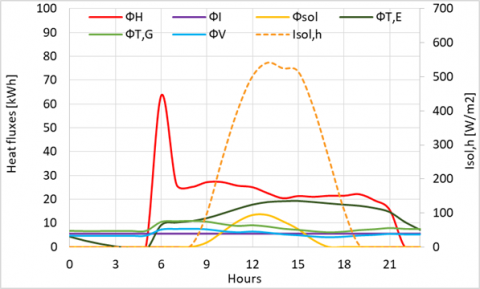(b) 1946-60

## 53.png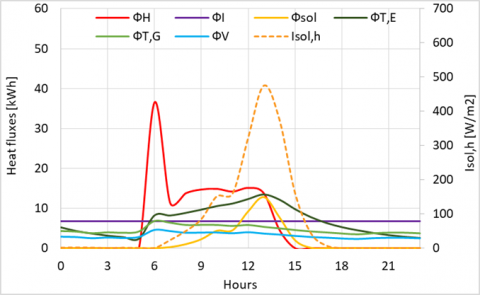(c) 1961-70

## 54.png(d) 1971-80

Figure 4. Heat flux components for a typical day: (a)-(b) 22/2/2014, Tae = 6.24℃; (c)-(d) 24/10/2013, Tae = 16.3℃

Figure 4 shows the results of the energy balance model for a number of constant air changes per hour of 0.5 h-1 over 24 hours for two typical days: February 22nd 2014 with Tae equal to 6.24℃ and October 24th 2013 with Tae equal to 16.3℃. With this type of representation, it is possible to assess the weight of each energy balance component. It is emerged that there is a significant rate of heat flow loss for ΦT,E and ΦT,G and the typical behavior of heat realized by the heating system ΦH with a peak at 6 a.m.

4.2 Temperatures of the three thermodynamic systems

The temperatures of the three thermodynamic systems (B, E and G) are shown in Figure 5, with the outside air temperature (in blue) and the solar irradiance (in yellow) on the right. The results are presented for the two typical days that is February 22nd 2014 and October 24th 2013.

It can emerge that the temperature of the building TB (in green) is quite constant during daytime and nighttime. Thermal comfort conditions, with an internal air temperature of 20 ± 2℃, are reached during the day, according to ISO 7730:2005 and EN 16789-1:2019. The three temperatures TB, TE and TG are obviously closer in October, because the outdoor air temperature Tae is somewhat warmer and there is less variation between temperatures.

## 51.png(a) 1919-45

## 52.png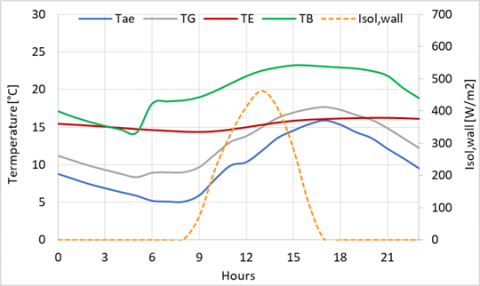(b) 1946-60

## 53.png(c) 1961-70

## 54.png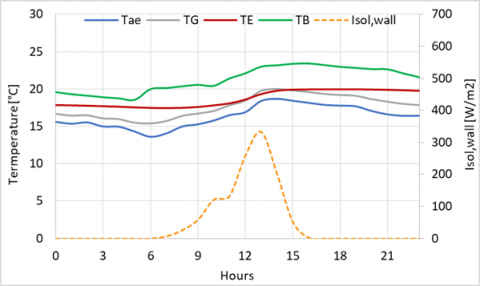(d) 1971-80

Figure 5. Building temperatures for a typical day: (a)-(b) 22/2/2014, Tae = 6.24℃; (c)-(d) 24/10/2013, Tae = 16.3℃

Figure 6 represented the temperature TB for the four selected buildings (with ach = 0.5 h-1) and considering different local climate conditions for four typical days: November 26th 2013, February 25th 2014, March 8th 2014, and March 13th 2014, with Tae equal to 5.3, 9.2, 12.5 and 15.3℃ respectively.

## 61.png(a) 1919-45

## 62.png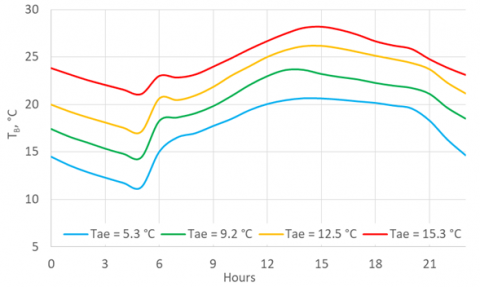(b) 1946-60

## 63.png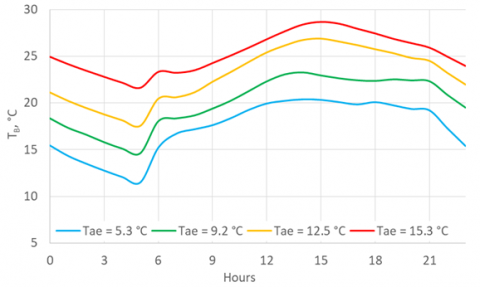(c) 1961-70

## 64.png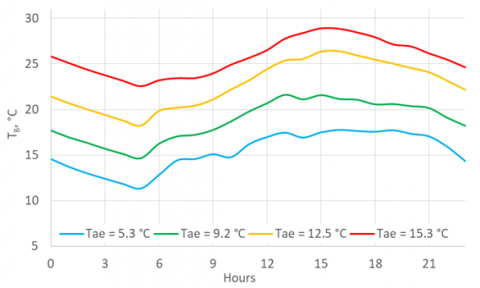(d) 1971-80

Figure 6. Building temperatures for four typical days: 26/11/2013, 25/2/2014, 8/3/2013, 13/3/2014

## 71.png(a) daytime correlations

## 72.png(b) nighttime correlations

Figure 7. Correlations between TB and Tsol-air throughout the daytime, from 6 a.m. to 9 p.m.; TB and Tae during the nighttime, from 10 p.m. to 5 a.m

From this comparison, it is possible to observe that the daytime and nighttime temperatures of TB are quite stable, and this trend depends on the characteristics of the buildings and on the local external climate conditions. Subsequently, the dynamic model was optimized by assuming the obtained correlations between the local climate conditions (Tair and Tsol-air) –distinguishing daytime and nighttime– and the temperatures of the building, TB (Figure 7).

4.3 Sensitivity analysis

In order to define the model and verify that the results were consistent, a sensitivity analysis was carried out on the thermal model in which calculated consumptions were simulated without considering some of the thermal balance components. In particular, the consumptions of the following scenarios were compared:

- S0: with all the components,

- S1: S0 without ventilation dispersions,

- S2: S0 without internal gains and transmitted solar flows and

- S3: S0 without dispersions due to ventilation and internal gains.

Table 7 and Figure 8 show the results of this analysis. The reference scenario is S0 (in red in Figure 9); it can be seen that consumption only increases for all construction periods, if both the internal and solar inputs are removed, while it decreases if the ventilation losses are removed (as it was logical to expect).

Table 7 also shows the percentage shares of increase or decrease in annual consumption according to the period of construction of the buildings under the same local climatic conditions.

## 81.png(a) 1919-45

## 82.png(b) 1946-60

## 83.png(c) 1961-70

## 84.png(d) 1971-80

Figure 8. Building consumptions (in Wh) for the 4 archetypes and the different scenarios

Table 7. Energy consumption variations obtained by changing the heat balance components

 19-45 46-60 61-70 71-80 $\phi_{I}$ $\tau_{G} \cdot I_{\text {sol}}$ $\dot{m}_{a}$ S0 1.00 1.00 1.00 1.00 Y Y Y S1 0.70 0.69 0.71 0.74 Y Y N S2 1.12 1.31 1.17 1.18 N Y Y S3 0.82 1.00 0.88 0.92 N Y N

4.4 Model validation

The hourly space heating consumption of the residential buildings was calculated by applying the model with the temperature correlations. The graphs below show the results of the comparison between the simulated and measured consumptions for the 2013-14 heating season for the four selected buildings.

Figure 9 shows the results of a comparison between the simulated and measured daily thermal consumption. A very good accuracy can be observed for buildings built in the 1949-45, 1946-60 and 1961-70 periods. The accuracy decreases for buildings constructed in the 1971-80 period. This problem arose also because this sample is composed of a limited number of buildings and further investigations will be made in the future to optimize the precision of the model.

## 91.png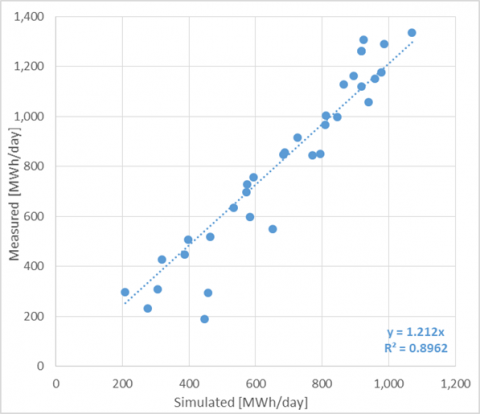(a) 1919-45

## 92.png(b) 1946-60

## 93.png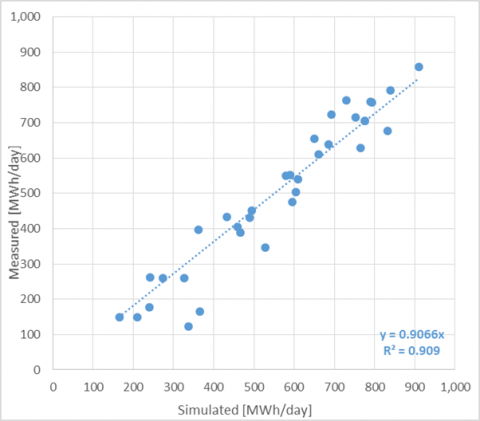(c) 1961-70

## 94.png(d) 1971-80

Figure 9. Comparison between the simulated and measured space heating consumptions

5. Conclusions

The objective of this work has been to develop an engineering model that can be used for the evaluation of the heating consumption of buildings in European cities. This model should be able to use existing spatial databases, and, for this reason, the model was associated with a GIS tool. Starting from building scale consumption models, the energy-balance equations have been simplified so that data can be found in the technical map for all buildings in a city. In addition, variables have been calculated so that the urban features around each building, that influence its consumption, can be described.

The energy-balance model was initially applied using a spreadsheet to a limited group of buildings A specific code was then implemented using thermal-electrical analogy with a scientific computation and simulation software in order to extend the use of the model to all the buildings considered in the analysis. The results of this work show that the model works very well with buildings that have high consumptions. However, the accuracy of the model decreases for newer buildings due also to a smaller number of buildings with these characteristics.

The thermal-electrical analogy is suitable to manage a large amount of data with less time-consuming, to evaluate the specific contribution of the heat flows, and compare the heat flows among the elements of the systems.

Moreover, this analogy can help to solve the energy balance if new elements are added to the system or if reference days are considered and the system is assumed to work under periodic in steady state conditions.

Nomenclature
 ach Number of air changes per hour, h-1 A area, m2 b correction factor for unconditioned spaces c specific heat capacity, Jkg-1K-1 C heat (thermal) capacity, JK-1 DH district heating, - EP energy performance index, kWh m-2K-1 EPC Energy performance certificate F reduction/view factor, - h surface coefficient of heat transfer, Wm-2K-1 H/W urban canyon height-to-width ratio, - I solar irradiance, Wm-2 R thermal resistance, m2KW-1 S floor surface, m2 SVF sky view factor, - t time, s T temperature, °C or K TS thermodynamic system U thermal transmittance, Wm-2K-1 V volume, m3 Greek symbols α absorption coefficient of solar radiation, - ε emissivity η system efficiency for space heating, - ρ density, kgm-3 λ conductivity, Wm-1K-1 ξ shadow quota, - τ total solar energy transmittance, - Φ heat flow rate, thermal power, W ψ solar height, ° Subscripts ae external air B building E envelope e external g gross G glazing H heating int internal k building element n net se external surface sol solar T transmission v ventilation w windows
References

 Rosenow, J., Cowart, R., Bayer, E., Fabbri, M. (2017). Assessing the European Union’s energy efficiency policy: Will the winter package deliver on ‘Efficiency First’? Energy Research & Social Science, 26: 72-79. https://doi.org/10.1016/j.erss.2017.01.022

 Hedegaard, R.E., Kristensen, M.H., Pedersen, T.H., Brun, A., Petersen, S. (2019). Bottom-up modelling methodology for urban-scale analysis of residential space heating demand response. Applied Energy, 242: 181-204. https://doi.org/10.1016/j.apenergy.2019.03.063

 Mutani, G., Todeschi, V. (2019). An urban energy atlas and engineering model for resilient cities. International Journal of Heat and Technology, 37(4): 936-947. https://doi.org/10.18280/ijht.370402

 Mutani, G., Todeschi, V. (2020). Building energy modeling at neighborhood scale. Energy Efficiency. https://doi.org/10.1007/s12053-020-09882-4

 Abbasabadi, N., Ashayeri, M. (2019). Urban energy use modeling methods and tools: A review and an outlook. Building and Environment, 161: 106270. https://doi.org/10.1016/j.buildenv.2019.106270

 Chen, Y., Hong, T., Piette, M.A. (2017). Automatic generation and simulation of urban building energy models based on city datasets for city-scale building retrofit analysis. Applied Energy, 205: 323-335. https://doi.org/10.1016/j.apenergy.2017.07.128

 Mutani, G., Todeschi, V., Beltramino, S. (2020). Energy consumption models at urban scale to measure energy resilience. Sustainability, 12(14): 5678. https://doi.org/10.3390/su12145678

 Mutani, G., Todeschi, V., Matsuo, K. (2019). Urban heat island mitigation: A GIS-based model for Hiroshima. Instrumentation Mesure Métrologie, 18(4): 323-335. https://doi.org/10.18280/i2m.180401

 Chen, Q., Fu, R.H., Xu, Y.C. (2014). Electrical circuit analogy for heat transfer analysis and optimization in heat exchanger networks. Applied Energy, 139: 81-92. https://doi.org/10.1016/j.apenergy.2014.11.021

 Fracastoro, G.V., Mutani, G., Perino, M. (2002). Experimental and theoretical analysis of natural ventilation by windows opening. Energy and Buildings, 34(8): 817-827. https://doi.org/10.1016/S0378-7788(02)00099-3

 Alhamwi, A., Medjroubi, W., Vogt, T., Agert, C. (2017). GIS-based urban energy systems models and tools: Introducing a model for the optimisation of flexibilisation technologies in urban areas. Applied Energy, 191: 1-9. https://doi.org/10.1016/j.apenergy.2017.01.048

 Capizzi, G., Lo Sciuto, G., Cammarata, G., Cammarata, M. (2017). Thermal transient simulations of a building by a dynamic model based on thermal-electrical analogy: Evaluation and implementation issue. Applied Energy, 199: 323-334. https://doi.org/10.1016/j.apenergy.2017.05.052

 Fraisse, G., Viardot, C., Lafabrie, O., Achard, G. (2002). Development of a simplified and accurate building model based on electrical analogy. Energy & Buildings, 34(10): 1017-1031. https://doi.org/10.1016/S0378-7788(02)00019-1

 Robinson, D., Haldi, F., Kampf, J.H., Leroux, P., Perez, D., Rasheed, A., Wilke, U. (2009). CITYSIM: Comprehensive micro-simulation of resource flows for sustainable urban planning. Eleventh International IBPSA Conference, Glasgow, Scotland.

 Boghetti, R., Fantozzi, F., Kämpf, J.H., Mutani, G., Salvadori, G., Todeschi, V. (2019) Building energy models with morphological urban-scale parameters: A case study in Turin. In Book of Proceedings of BSA 2019, pp. 131-139.

 AA. VV. - TABULA Typology Approach for Building Stock Energy Assessment – Webtool, http://webtool.building-typology.eu/#bm, accessed on January 5th 2020.

 Mutani, G., Gabrielli, C., Nuvoli, G. (2020). Energy performance certificates analysis in piedmont region (IT). A New Oil Field Never Exploited Has Been Discovered. Tecnica Italian-Italian Journal of Engineering Science, 64(1): 71-82. https://doi.org/10.18280/ti-ijes.640112